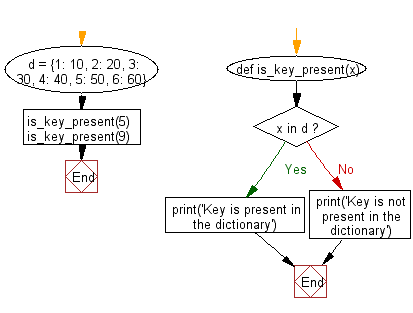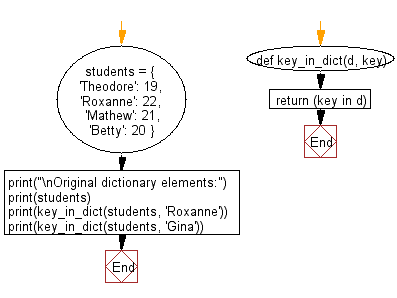﻿ Python: Check whether a given key already exists in a dictionary - w3resource# Python: Check whether a given key already exists in a dictionary

## Python dictionary: Exercise-4 with Solution

Write a Python program to check whether a given key already exists in a dictionary.

Sample Solution-1:

Python Code:

``````d = {1: 10, 2: 20, 3: 30, 4: 40, 5: 50, 6: 60}
def is_key_present(x):
if x in d:
print('Key is present in the dictionary')
else:
print('Key is not present in the dictionary')
is_key_present(5)
is_key_present(9)
```
```

Sample Output:

```Key is present in the dictionary
Key is not present in the dictionary
```

Flowchart:## Visualize Python code execution:

The following tool visualize what the computer is doing step-by-step as it executes the said program:

Sample Solution-2:

Python Code:

``````def key_in_dict(d, key):
return (key in d)
students = {
'Theodore': 19,
'Roxanne': 22,
'Mathew': 21,
'Betty': 20
}
print("\nOriginal dictionary elements:")
print(students)
print(key_in_dict(students, 'Roxanne'))
print(key_in_dict(students, 'Gina'))
```
```

Sample Output:

```Original dictionary elements:
{'Theodore': 19, 'Roxanne': 22, 'Mathew': 21, 'Betty': 20}
True
False
```

Flowchart:## Visualize Python code execution:

The following tool visualize what the computer is doing step-by-step as it executes the said program:

Python Code Editor:

Have another way to solve this solution? Contribute your code (and comments) through Disqus.

What is the difficulty level of this exercise?

Test your Programming skills with w3resource's quiz.

﻿

## Python: Tips of the Day

Invokes the provided function after ms milliseconds:

Example:

```from time import sleep

def tips_delay(fn, ms, *args):
sleep(ms / 1000)
return fn(*args)
print(tips_delay(
lambda x: print(x),
1000,
'w3r'
))
```

Output:

```w3r
None
```